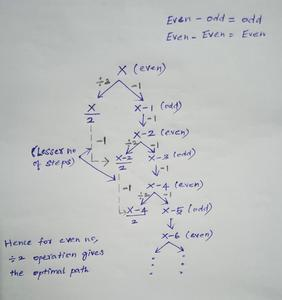Open In App

# Minimize steps to reach K from 0 by adding 1 or doubling at each step

Given a positive integer K, the task is to find the minimum number of operations of the following two types, required to change 0 to K:

• Add one to the operand
• Multiply the operand by 2.

Examples:

Input: K = 1
Output:
Explanation:
Step 1: 0 + 1 = 1 = K
Input: K = 4
Output:
Explanation:
Step 1: 0 + 1 = 1,
Step 2: 1 * 2 = 2,
Step 3: 2 * 2 = 4 = K

Approach:

• If K is an odd number, the last step must be adding 1 to it.
• If K is an even number, the last step is to multiply by 2 to minimize the number of steps.
• Create a dp[] table that stores in every dp[i], the minimum steps required to reach iProof:

• Let us consider an even number X
• Now we have two options:
i) X-1
ii)X/2
• Now if we choose X-1:
-> X-1 is an odd number , since X is even
-> Hence we choose subtract 1  operation and we have X-2
->Now we have X-2, which is also an even number , Now again we have two options either to subtract 1 or to divide by 2
->Now let us choose divide by 2 operation , hence we have ( X – 2 )/2 => (X/2) -1
• Now if we choose X/2:
-> We can do the subtract 1 operation to reach (X/2)-1
• Now let us consider sequence of both the cases:
When we choose X-1 : X -> X-1 -> X-2 -> (X/2)-1 [ totally three operations ]
When we choose X/2 : X -> X/2 -> (X/2)-1 [ totally two operations ]This diagram shows how choosing divide operation for even numbers leads to optimal solution recursively

• Hence we can say that for a given even number , choosing the divide by 2 operation will always give us the minimum number of steps
• Hence proved

Below is the implementation of the above approach:

## C++

 // C++ program to implement the above approach#include using namespace std; // Function to find minimum operationsint minOperation(int k){    // vector dp is initialised    // to store the steps    vector<int> dp(k + 1, 0);     for (int i = 1; i <= k; i++) {         dp[i] = dp[i - 1] + 1;         // For all even numbers        if (i % 2 == 0) {            dp[i]                = min(dp[i],                      dp[i / 2] + 1);        }    }    return dp[k];} // Driver Codeint main(){    int K = 12;    cout << minOperation(k);}

## Java

 // Java program to implement the above approachclass GFG{     // Function to find minimum operationsstatic int minOperation(int k){         // dp is initialised    // to store the steps    int dp[] = new int[k + 1];     for(int i = 1; i <= k; i++)    {       dp[i] = dp[i - 1] + 1;               // For all even numbers       if (i % 2 == 0)       {           dp[i] = Math.min(dp[i], dp[i / 2] + 1);       }    }    return dp[k];} // Driver Codepublic static void main (String []args){    int K = 12;    System.out.print( minOperation(K));}} // This code is contributed by chitranayal

## Python3

 # Python3 program to implement the above approach # Function to find minimum operationsdef minOperation(k):         # dp is initialised    # to store the steps    dp =  * (k + 1)     for i in range(1, k + 1):        dp[i] = dp[i - 1] + 1         # For all even numbers        if (i % 2 == 0):            dp[i]= min(dp[i], dp[i // 2] + 1)     return dp[k] # Driver Codeif __name__ == '__main__':         k = 12         print(minOperation(k)) # This code is contributed by mohit kumar 29

## C#

 // C# program to implement the above approachusing System;class GFG{     // Function to find minimum operationsstatic int minOperation(int k){         // dp is initialised    // to store the steps    int []dp = new int[k + 1];     for(int i = 1; i <= k; i++)    {        dp[i] = dp[i - 1] + 1;                     // For all even numbers        if (i % 2 == 0)        {            dp[i] = Math.Min(dp[i], dp[i / 2] + 1);        }    }    return dp[k];} // Driver Codepublic static void Main(){    int K = 12;    Console.Write(minOperation(K));}} // This code is contributed by Nidhi_Biet

## Javascript

 

Output:

5

Time Complexity: O(k)
Auxiliary Space: O(k)